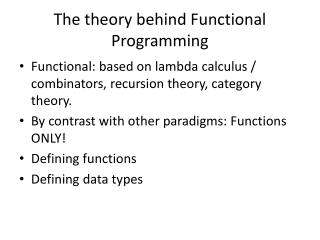# The theory behind Functional Programming - PowerPoint PPT PresentationDownload PresentationThe theory behind Functional Programming

The theory behind Functional Programming
Download Presentation## The theory behind Functional Programming

- - - - - - - - - - - - - - - - - - - - - - - - - - - E N D - - - - - - - - - - - - - - - - - - - - - - - - - - -
##### Presentation Transcript

1. The theory behind Functional Programming • Functional: based on lambda calculus / combinators, recursion theory, category theory. • By contrast with other paradigms: Functions ONLY! • Defining functions • Defining data types

2. Category Theory • Abstract view of functions as “arrows” with domains and codomains as their “source” and “target” objects • Things to watch: • How we compose arrows • Which arrows can be composed • How objects can be seen as identity arrows • How we connect categories with functors

3. Resources on Category Theory • http://en.wikipedia.org/wiki/Category_theory • https://www.google.com/search?q=Awodey++Category+Theory+&ie=utf-8&oe=utf-8&aq=t&rls=org.mozilla:en-US:official&client=firefox-a

4. Definition of a Category • Tw classes: Objects A,B,C,… and Arrowsf,g,h,… • For each arrow f : A->B, two objects A=dom(f) B=cod(f) • If f:A->B and f:B->C, their composition, denoted g . f : A->C exists • For each object A the arrow 1_A : A->A exists • For arrows f:A->B,g:B->C,h:C->D composition is associative: h . (g . f) = (h . g).f • For f:A->B, 1_A is a unit arrow: f . 1_A=f=1_B . f

5. Examples of Categories • Finite sets • Groups with group homomorphisms • Vector spaces with linear mappings • Graphs with graph homomorphisms • Topological spaces with continous functions • Posets and monotone functions

6. Functional programming seen as a category • Objects: monomorphic data types: Integer, Boolean, records of employees • Arrows: functions between data types • Id:: a -> a provides 1_a for each type a • “.” provides composition – and it is defined if types match • Function composition is associative

7. Functors • a (covariant) functorF : C → D: • for each object x in C, an object F(x) in D • for each arrow f : x → y in C, an arrow F(f) : F(x) → F(y), • such that the following two properties hold: • For every object x in C, F(1x) = 1F(x); • For all morphismsf : x → y and g : y → z, F(g ∘ f) = F(g) ∘ F(f). • Of special interest: endofunctors F:C->C

8. The Initial Algebra Semantics for polymorphic data types (1) • F-algebra: can be used to represent data structures used in programming, such as lists and trees. Defined such that the following diagram commutes:

9. The Initial Algebra Semantics for polymorphic data types (2) • the functor F: Set -> Set that sends a set X to 1+X • + denotes the usual coproduct (also called “sum”) given by disjoint union, and 1 is a terminal object (i.e. any singleton set) • the set N of natural numbers together with the function succ : 1+N->N, is the + of the functions zero : 1->N (whose image is 0) and succ: 1+N->N (which sends an integer n to n+1)is an initial F-algebra

10. The Initial Algebra Semantics for polymorphic data types (3) • Types defined by using least fixed point construct with functor F can be regarded as an initial F-algebra, provided that parametricity holds for the type • Examples: data N = Zero | Succ N data List a = Nil | Cons a (List a) map provides the functor definition for lists: it preserves the structure while changing its payload

11. More Functors Folds